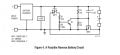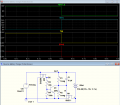Do not understand the reverse protection battery circuit.

ir_tronik

Joined Jul 12, 2017
16
Hi, I have read a reverse protection battery circuit using N-channel Mosfet. But, I do not understand clearly on how the operation works. Could someone explain to me on how the below circuit works? From during normal operation until the reverse polarity happened.Here is the source of this circuit in page 2:
https://www.analog.com/media/en/technical-documentation/application-notes/AN-171_AN-1568.pdf

AnalogKid

Joined Aug 1, 2013
9,631
The app not you linked to explains the function of each component. What parts, specifically, are not clear to you?

ak

crutschow

Joined Mar 14, 2008
28,545
Below is the LTspice simulation of the circuit.
The circuit operation depends upon the charger having some internal impedance (shown by the Rser=1).

For the first half-second of simulation the charger is charging normally with the battery voltage (green trace) at plus 12V.
P-MOSFET M2 is off (gate voltage more positive than the source) with 0V at its drain (blue trace) and thus Q1 is also off, allowing R1 to apply positive voltage to N-MOSFET M1's gate (yellow trace) and turning it on.
This allows the battery to have normal charging current (red trace)

When the battery voltage is reversed at the 0.5s point (green trace), the positive voltage from the battery at M2's source turns it on (blue trace).
This turns on Q1, removing M1's gate voltage (yellow trace) and stopping the charging current (red trace).
Note there is an unavoidable, short high spike of current when the battery is reversed, which is normal and should do no damage to the charger.ir_tronik

Joined Jul 12, 2017
16
The app not you linked to explains the function of each component. What parts, specifically, are not clear to you?

ak
the C1 part. It tell us about the it provides the ultra fast charge pump

ir_tronik

Joined Jul 12, 2017
16
Below is the LTspice simulation of the circuit.
The circuit operation depends upon the charger having some internal impedance (shown by the Rser=1).

For the first half-second of simulation the charger is charging normally with the battery voltage (green trace) at plus 12V.
P-MOSFET M2 is off (gate voltage more positive than the source) with 0V at its drain (blue trace) and thus Q1 is also off, allowing R1 to apply positive voltage to N-MOSFET M1's gate (yellow trace) and turning it on.
This allows the battery to have normal charging current (red trace)

When the battery voltage is reversed at the 0.5s point (green trace), the positive voltage from the battery at M2's source turns it on (blue trace).
This turns on Q1, removing M1's gate voltage (yellow trace) and stopping the charging current (red trace).
Note there is an unavoidable, short high spike of current when the battery is reversed, which is normal and should do no damage to the charger.

View attachment 253863
Yes, that helps me understand it. Thank you. But, what is the function of the C1?

crutschow

Joined Mar 14, 2008
28,545
what is the function of the C1?
It discharges rapidly when the battery is reversed due to the negative voltage now being applied to the positive output, which reduces the turn off time of the N-MOSFET.

AnalogKid

Joined Aug 1, 2013
9,631
R3 and R4 form a Thevenin equivalent voltage source of 1/2 the battery charger circuit output voltage, through a parallel combination of the two resistors. For this explanation, let's assume the charger is 12 V, and the gate voltage is 6 V through 500 K.

There are several combinations of conditions that this circuit might see. the charger circuit can be powered or unpowered, and the connected battery can be fully charged, partially charged, or completely discharged. That works out to six possible circuit conditions, and one of them is the worst. When the circuit is powered up and a fully charged battery is connected backwards, the power dissipation in MN1 is the highest possible of all conditions. To limit this power dissipation, it must be turned off as fast as possible.

C1 is not very big, but it is way bigger than the gate capacitance of MN1. When the battery is connected backwards, the negative end is connected to C1 and the positive end is connected to the MN1 drain. This applies a large negative voltage to the gate, which will turn off the FET. However, the FET's gate capacitance has been charged up through R3||R4. Without C1, that capacitance has to discharge through R3||R4 before the FET turns off, and that will take at least one time constant, or 50 milliseconds. During that time, the FET is a dead short across the battery, trying to suck down a lot of amps. This can damage both the FET and the battery.

The voltage across a capacitor cannot change instantaneously. In a 12 V system, the gate normally rests at 6 V, so that is the voltage across C1 when the batter is connected. In less than a microsecond, C1 is yanked 12 volts negative, and the gate voltage (Vgs) dives from +6 V to -6 V. C1 is big enough to overpower the MN1 gate capacitance, so the FET turns off very quickly. This action where a capacitor is charged up to a voltage, and then reconnected to add or subtract that voltage with something else, is called a charge pump.

https://en.wikipedia.org/wiki/Charge_pump

ak